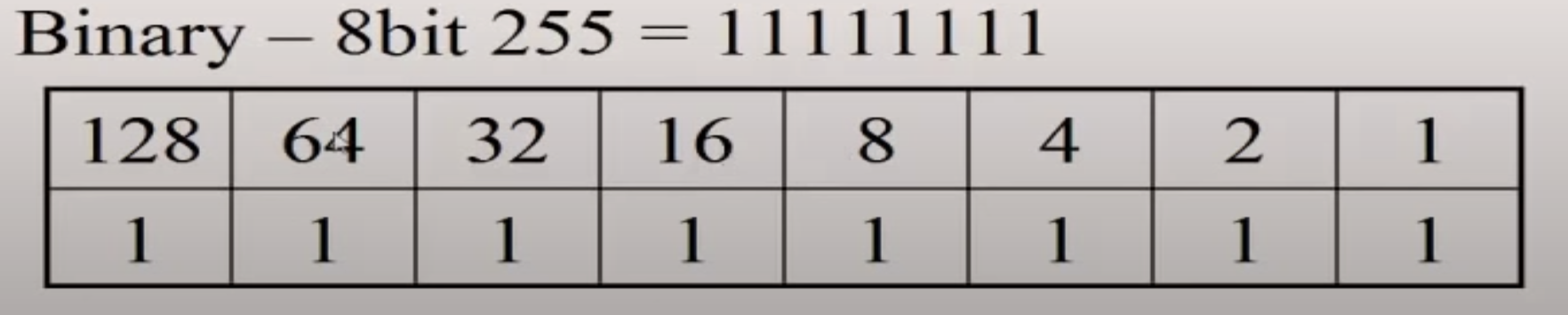# Binary

Binary data can come in many different formats. For example, let’s consider a binary sequence representing a byte of data: 01110110. If this binary sequence represented a string in English using the ASCII encoding standard, it would be the letter v. However, if our computer was processing an image, that binary sequence could contain information about the color of a pixel.

• The computer knows to process them differently because the bytes are encoded differently.
• Byte encoding is the format of the byte (e.g. ascii, UTF-8).
• ex. if it's encoded in UTF-8 (as is the case in Node buffers), then we know it's a number, letter or symbol.

# Bits and Bytes

• Bit - a zero or 1
• portmanteau of "binary digit"
• Byte - an atomic unit of digital information. From a memory standpoint, it is the smallest unit that can be stored and read.
• Though 8 bits make up a byte, there is no reason for that number aside from the benefits gained by raising that number to the power of 2.
• There have been many other instances of varying-bit bytes, such as 10-bit bytes, 4-bit bytes etc.
• 1 octet equals 1 byte (as long as the bytes exist in an 8-bit system, which is almost always)
• by definition, binary numbers ending in 0 are even, and those ending in 1 are odd.

## Bit

the number 255 represents 8 bits.

• Therefore, it is the largest binary number that can be represented by 8 individual bits.
• in binary, it is 11111111

### Conversion

• the image below shows how the placement of the bit determines its numerical value on the whole.
- just like in base 10, each placement to the left multiplies the number by 10, in base 2, each placement to the left multiplies the number by 2
- if we add up the value of each numerical position (128, 64...), we will get 255• This chart above can be used to convert to/from binary format
• ex. if we want to represent 135 in binary, we just need to add each slot, with the biggest fitting number first. In this case, the 8th slot, the 3rd slot, the 2nd slot and the 1st slot (or, 10000111)

• a bit can be stored by any device that can exist in one of 2 possible states
• ex. lightswitch, presence/absense of a hole in a punchcard, thickness of barcode line, presence of a microscopic pit on a CD ROM
• therefore, any of these methods can be used to represent 0's and 1's.
• in the case of modern computers, that bit is stored by way of: electrical pulse, or no electrical pulse

### Bit field

• a type of data structure that directly stores bits